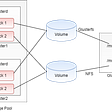# Python Programming — Function

`def hello():    print('Hello world !')hello()hello()hello()`
`Hello worldHello worldHello world`
`def hello(nama):    print('Hello %s!' % nama)def print_max(a, b):    if a > b:        print('%s is the maximum value' % a)    elif a == b:        print('%s equals %s' % (a, b))    else:        print('%s is the maximum value' % b)hello('World')  hello('Indonesian People')print_max(100, 100)x = 5y = 3print_max(x, y)`
`Hello World!Hello Indonesian People!100 equals 1005 is the maximum value`
• Function that returns no value
`def areaTriangle(a,t):    area = a*t/2    print('Area of triangle with base', a,          'and height', t,          'is', area)base = 10height = 20areaTriangle(base,height)`
`Area of triangle with base 10 and height 20 is 100.0`
• Function that returns value
`def areaTriangle(a,t):    area = a*t/2    return areabase = 9height = 21print('Area of triangle with base', base,' and high', height,'is', areaTriangle(base,height))`
`Area of triangle with base 9  and high 21 is 94.5`
`x = 50def function(x):    print('x = ', x)    x = 2    print('change local variable x = ', x)function(100)print('x value is still %s' % x)`
`x =  100change local variable x =  2x value is still 50`
`x = 50def function():    print('x = ', x)def function2():    x = 100 # write to local variable    print('x = ', x)def function3():    global x    x = 100    print('x = ', x)function()print('value x = ', x)function2()print('value x = ', x)function3()print('value x = ', x)`
`x =  50value x =  50x =  100value x =  50x =  100value x =  100`

--

--

--

## More from Nabil Makarim

NIM (K3521056), EMAIL : bluerejet1330@gmail.com, UNIVERSITAS SEBELAS MARET

Love podcasts or audiobooks? Learn on the go with our new app.

## Community Development Grants## Remix in VSCode now has DEPLOY & RUN## A Complete 12-Week Course to Learn Web Scraping in Python for Free## On becoming a professional web developer in one year## SOAP API Testing Using Postman## 7 Lessons for Self-Taught Developers## GlusterFS Basics, Installation and Usage## Nabil Makarim

NIM (K3521056), EMAIL : bluerejet1330@gmail.com, UNIVERSITAS SEBELAS MARET

## Memory ecosystem of python: Immutable and Mutabel Objects(a.k.a eveything is object)## For loop in python## Walrus Operator Explained [Python]## Cool way to print out Python keywords in Python